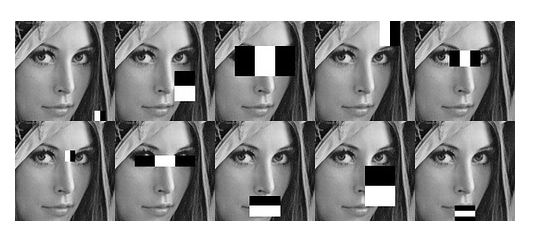/  Deep Learning Interview questions and answers   /  What do you mean by Haar cascades in Computer Vision? What are the different Haar Cascades available in Computer Vision?## What do you mean by Haar cascades in Computer Vision? What are the different Haar Cascades available in Computer Vision?

A Haar-like feature considers neighboring rectangular regions at a specific location in a detection window, sums up the pixel intensities in each region and calculates the difference between these sums. This difference is then used to categorize subsections of an image. An example of this would be the detection of human faces. Commonly, the areas around the eyes are darker than the areas on the cheeks. One example of a Haar-like feature for face detection is therefore a set of two neighboring rectangular areas above the eye and cheek regions. The cascade classifier consists of a list of stages, where each stage consists of a list of weak learners. The system detects objects in question by moving a window over the image.

Each stage of the classifier labels the specific region defined by the current location of the window as either positive or negative – positive meaning that an object was found or negative means that the specified object was not found in the image. If the labelling yields a negative result, then the classification of this specific region is hereby complete and the location of the window is moved to the next location. If the labelling gives a positive result, then the region moves of to the next stage of classification. The classifier yields a final verdict of positive, when all the stages, including the last one, yield a result, saying that the object is found in the image.  A true positive means that the object in question is indeed in the image and the classifier labels it as such – a positive result.

A false positive means that the labelling process falsely determines, that the object is located in the image, although it is not. A false negative occurs when the classifier is unable to detect the actual object from the image and a true negative means that a nonobject was correctly classifier as not being the object in question. In order to work well, each stage of the cascade must have a low false negative rate, because if the actual object is classified as a non-object, then the classification of that branch stops, with no way to correct the mistake made. However, each stage can have a relatively high false positive rate, because even if the n-th stage classifies the non-object as actually being the object, then this mistake can be fixed in n+1-th and subsequent stages of the classifier.

### The algorithm has four stages:

Haar Feature Selection

Creating Integral Images